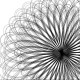Don't Miss

# The ultimate question

By on 30/11/2019If you are a reader of the Hitchhiker’s guide to the galaxy, you probably know that 42 is the answer to the Ultimate Question of Life, the Universe, and Everything. The choice of the number by Douglas Adams was quite random, excluding the simple fact that the number liked the writer. Yet the 42 was the protagonist of a recent news related to one of the open problems of mathematics:

Is there a number that is not 4 or 5 modulo 9 and that cannot be expressed as a sum of three cubes?

To find an answer to this question, mathematicians used numerical methods. In particular, Andreas-Stephan Elsenhans and Jorg Jahnel  using a particular vector space , searched solutions of the following diophantine equation:$x^3 + y^3 + z^3 = n$

for$n \leq 1000$ with$\displaystyle \max (|x|,|y|,|z|) \, \displaystyle \textless \, 10^{14}$. This method was later developed by Sander Husiman  to$\displaystyle \max (|x|,|y|,|z|) \, \displaystyle \textless \, 10^{15}$. In the end all numbers, except for 33 and 42, below 100 that are not 4 or 5 module 9 have solutions.
The cubic decomposition of these two numbers come in 2019. In both cases, the protagonist was Andrew Booker . In the case of 33 the solution arrives in march:$\begin{array}{c} (-80538738812075974)^3 \, + \, (-8778405442862239)^3 \, + \\+ \, (-2736111468807040)^3 = 33\end{array}$

It’s interesting to observe that Booker, in abstract, write that he was inspired by a Numperphile‘s youtube video:

The solution for 42 arrived at the beginning of september:$\begin{array}{c} (8866128975287528)^3 \, + \, (80435758145817515)^3 \, + \\+ \, (12602123297335631)^3 = 42\end{array}$

In this case Booker obtains his result in collaboration with Andrew Sutherland: in this way the list of all numbers less than 100 that are not 4 or 5 module 9 is completed.
Now, in the list of numbers between 100 and 1000, the numbers without a cubic decomposition are: 114, 165, 390, 579, 627, 633, 732, 921, e 975.

1. Forgive me for the excessive simplification.
2. Elsenhans, Andreas-Stephan; Jahnel, Jörg (2009), New sums of three cubes, Mathematics of Computation, 78 (266): 1227–1230, doi:10.1090/S0025-5718-08-02168-6
3. Huisman, Sander G. (2016), Newer sums of three cubes, arXiv:1604.07746
4. Booker, A.R. Cracking the problem with 33. Res. number theory (2019) 5: 26. doi:10.1007/s40993-019-0162-1 (arXiv